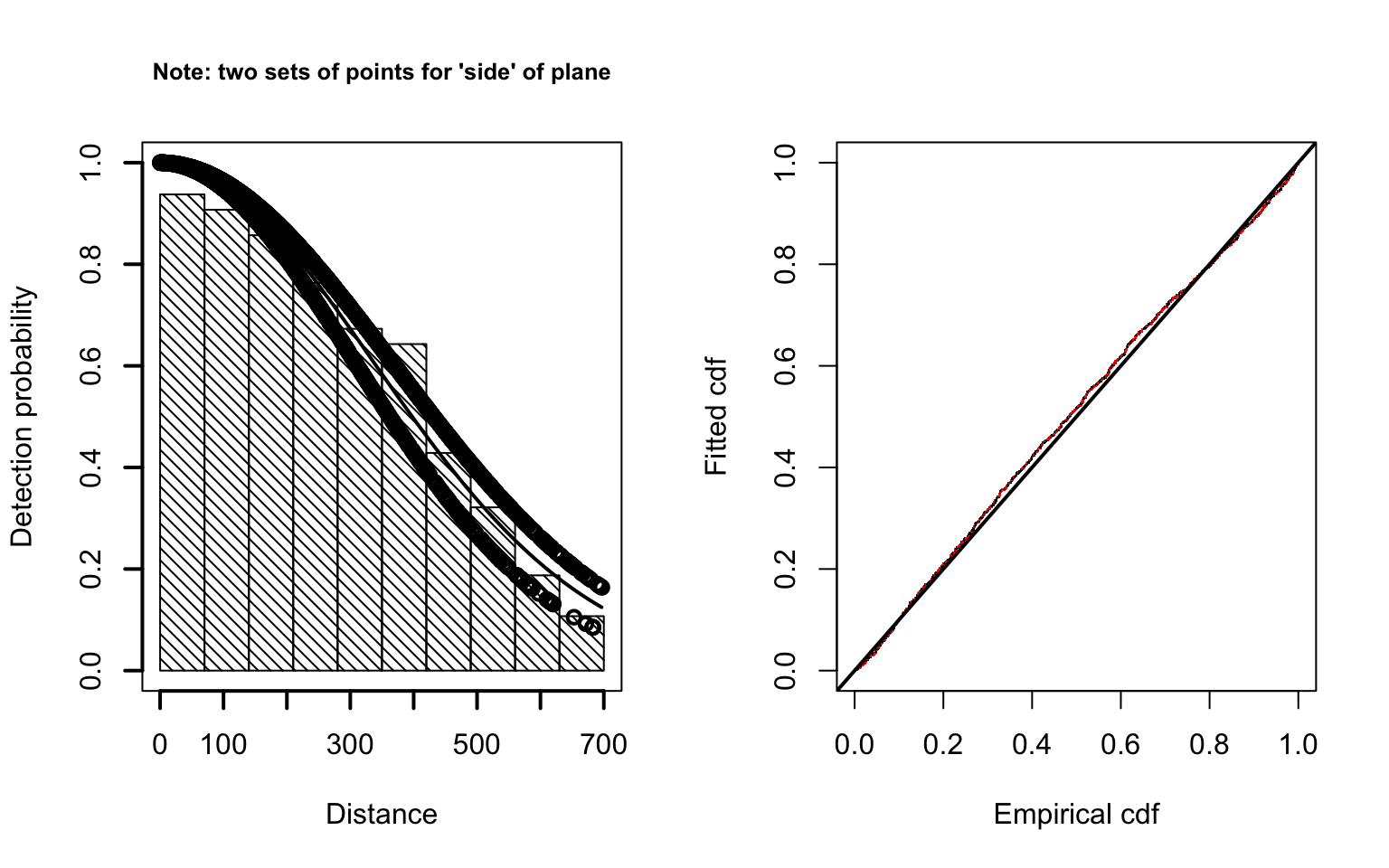The file crabbieMCDS.csv has data on crabeater seals, as if you had entered these data yourself into a spreadsheet.

This short exercise guides you through the import of those data into R, and fits a simple half-normal detection function examining the possible improvement of the model by incorporating a side of plane covariate.

library(Distance)
head(cr.covariate)
##     Study.area Region.Label    Area Sample.Label Effort distance size side
## 1 Nominal_area            1 1000000        99A21  59.72   144.49    1    R
## 2 Nominal_area            1 1000000        99A21  59.72   125.16    1    L
## 3 Nominal_area            1 1000000        99A21  59.72   421.40    1    L
## 4 Nominal_area            1 1000000        99A21  59.72    55.70    1    R
## 5 Nominal_area            1 1000000        99A21  59.72   288.52    1    R
## 6 Nominal_area            1 1000000        99A21  59.72     1.56    1    L
##     exp fatigue gscat vis glare ssmi altitude obsname
## 1   0.0   61.90     1   G     N   79    43.06      YH
## 2 211.7   62.61     1   G     N   79    43.06      MF
## 3   0.0   62.86     1   G     N   79    43.06      MH
## 4   0.0   62.96     1   G     N   79    42.84      YH
## 5   0.0   63.21     1   G     N   79    42.84      YH
## 6 211.7   63.45     1   G     N   79    42.84      MF

Noting that the data have been read into R appropriately, we are ready to fit a detection function.

Because we are using ddf() to perform model fitting, it expects to have double observer data. We are treating these data as if they were not employing the double observer configuration. Hence a function checkdata() comes to our aide to configure our data into the appropriate format for ddf().

Note that side of plane is assigned characters (L/R) in our data. Before using side of plane as a covariate, we need to tell R that side of plane takes on discrete values, hence characters are acceptable for use; this happens with the factor() function.

cr.covariate.fixed <- Distance:::checkdata(cr.covariate)
cr.covariate.fixed$side <- factor(cr.covariate.fixed$side)
cr.cov.ddf <- ddf(method="ds", dsmodel=~mcds(key="hn", formula=~side),
data=cr.covariate.fixed$data, meta.data=list(width=700)) summary(cr.cov.ddf) ## ## Summary for ds object ## Number of observations : 1740 ## Distance range : 0 - 700 ## AIC : 22305 ## ## Detection function: ## Half-normal key function ## ## Detection function parameters ## Scale Coefficients: ## estimate se ## (Intercept) 5.9037 0.03932 ## sideR -0.1747 0.05372 ## ## Estimate SE CV ## Average p 0.5826 0.01245 0.02137 ## N in covered region 2986.7380 78.92458 0.02643 Having fitted a detection function using side of plane as a covariate to the half-normal detection function, we would like to assess the fit of this function to our data. Two visual assessments are provided by the panels below: histogram and function on the left and QQ plot on the right. par(mfrow = c(1, 2)) plot.title <- "Note: two sets of points for 'side' of plane" plot(cr.cov.ddf , lwd = 2, lty = 1, pch = 1, cex = 1, nc = 10, main = plot.title) ddf.gof.results <- ddf.gof(cr.cov.ddf, lwd = 2, lty = 1, pch = ".", cex = 0.5, col = c(1,2))We could also fit a detection function without the covariate and contrast the two models. cr.nocovar <- ddf(method="ds",dsmodel=~mcds(key="hn", formula=~1), data=cr.covariate.fixed$data, meta.data=list(width=700))
summary(cr.nocovar)
##
## Summary for ds object
## Number of observations :  1740
## Distance range         :  0  -  700
## AIC                    :  22314
##
## Detection function:
##  Half-normal key function
##
## Detection function parameters
## Scale Coefficients:
##             estimate      se
## (Intercept)    5.829 0.02686
##
##                      Estimate       SE      CV
## Average p              0.5846  0.01248 0.02135
## N in covered region 2976.4596 78.43287 0.02635

AIC score for model without covariate is 22314 and AIC score for model with side as a covariate is 22305 so the model that includes side as a covariate is preferred.

We could go on to produce abundance estimates from our preferred model using dht(cr.cov.ddf) but leave that as an exercise for the reader.